# A meter stick is found to balance at the 49.7-cm mark when placed on a fulcrum....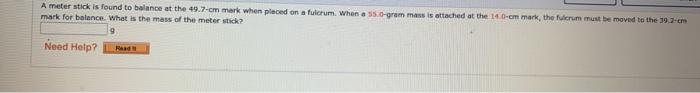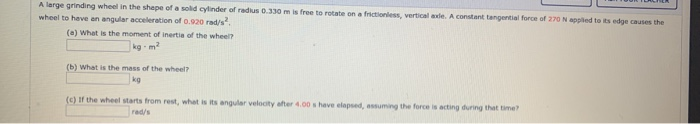A meter stick is found to balance at the 49.7-cm mark when placed on a fulcrum. When a 55.0-grammas is attached at the 14.0-cm mark the fulcrum must be moved to the 39.2- mark for balance. What is the mass of the meter stick Need Help? Raad
is free to rotate an a ctions , verticale. A constant tangential force of 270 N applied to its edge causes the A large grinding wheel in the shape of a solid cylinder ofredus 0.330 wheel to have an angular acceleration of 0.920 rad/s? (a) What is the moment of inertia of the wheel (b) What is the mass of the wheel? 4.00 have lapsed, assuming the force is acting during that time (c) of the wheel starts from rest, what is its angular velocity rad's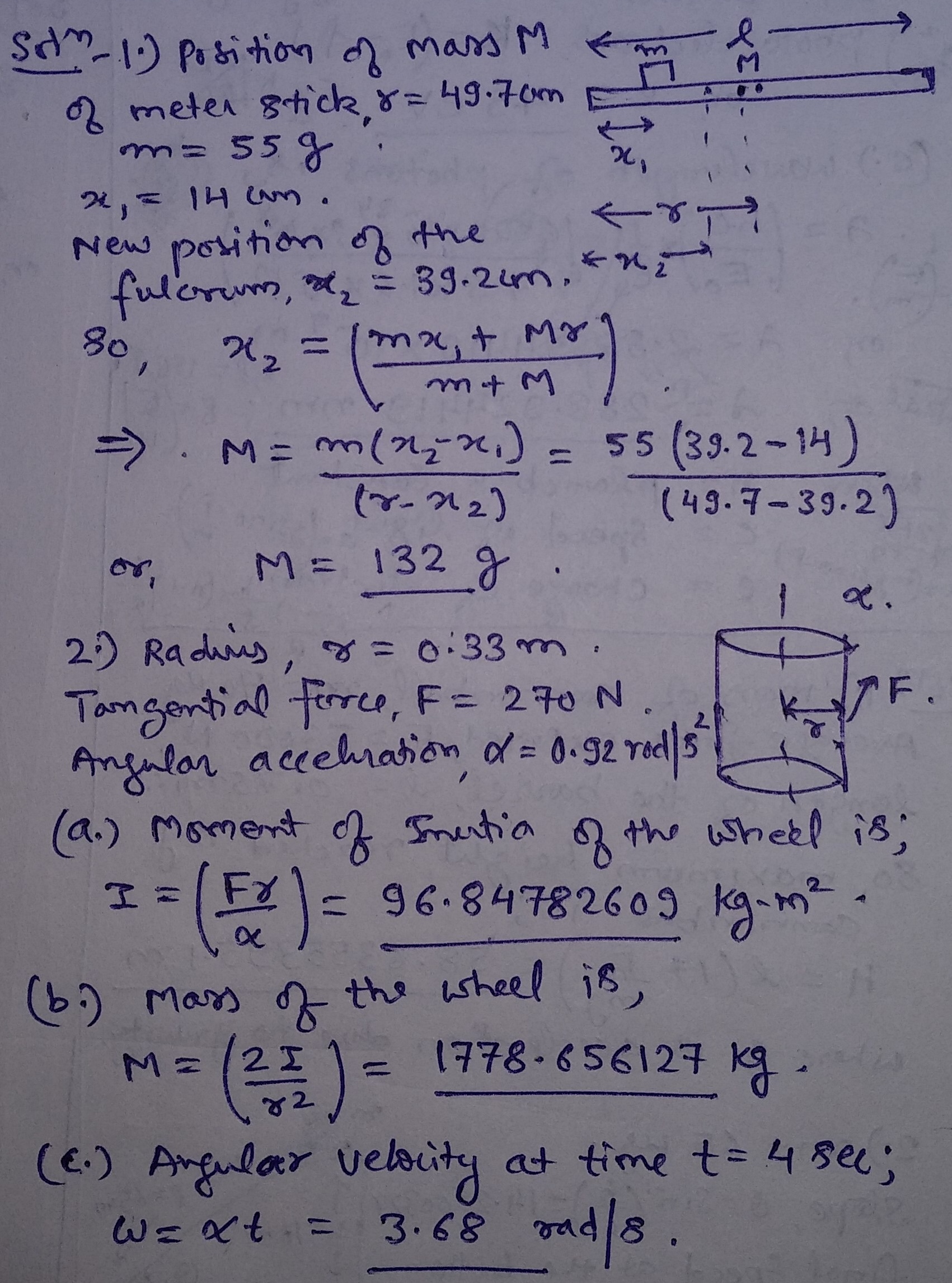#### Earn Coin

Coins can be redeemed for fabulous gifts.

Similar Homework Help Questions
• ### A meter stick is found to balance at the 49.7-cm mark when placed on a fulcrum....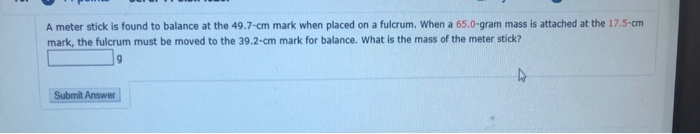A meter stick is found to balance at the 49.7-cm mark when placed on a fulcrum. When a 65.0 gram mass is attached at the 17.5 cm mark, the fulcrum must be moved to the 39.2-cm mark for balance. What is the mass of the meter stick? Submit Answer

• ### 4. The meter stick balances at the 49.7 cm mark when placed on the fulcrum, the...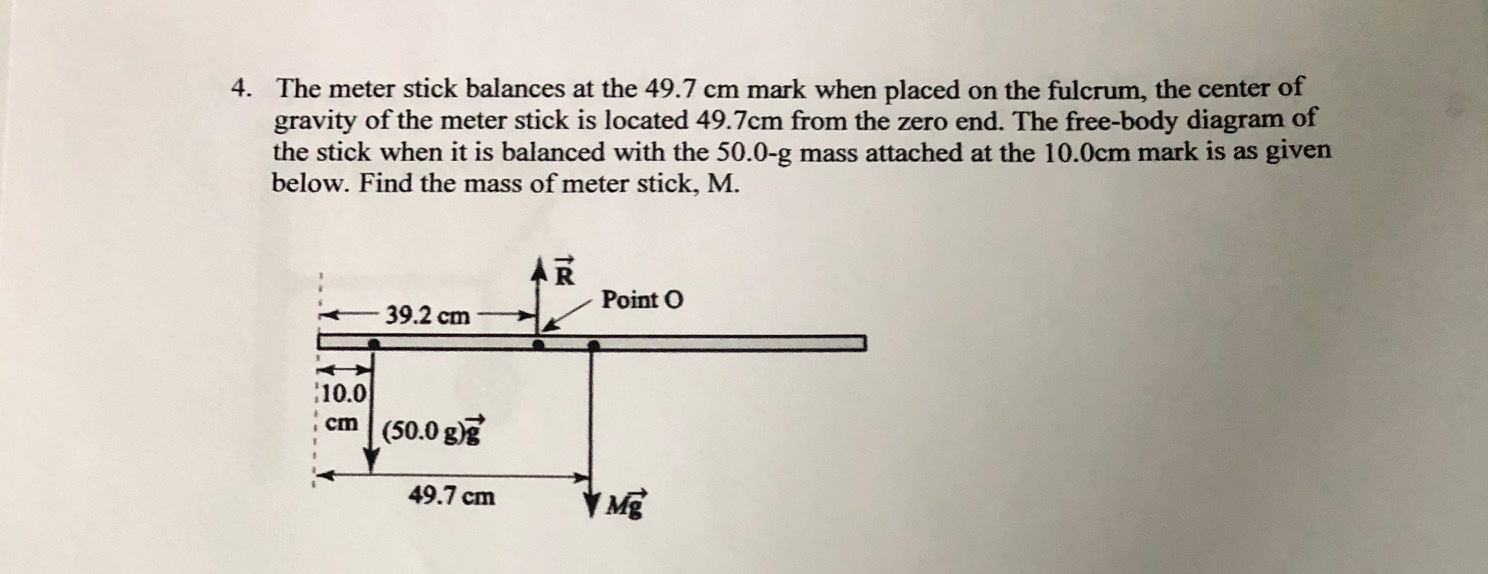4. The meter stick balances at the 49.7 cm mark when placed on the fulcrum, the center of gravity of the meter stick is located 49.7cm from the zero end. The free-body diagram of the stick when it is balanced with the 50.0-g mass attached at the 10.0cm mark is as given below. Find the mass of meter stick, M. AR Point O - 39.2 cm - 10.0 cm (50.0 g)? 49.7 cm 49.7 cm Me

• ### A meter stick is found to balance at the 50.0 cm mark when placed on a...

A meter stick is found to balance at the 50.0 cm mark when placed on a fulcrum. When a 0.100 kg mass is attached at the 10.0 cm mark, the fulcrum must be move to the 34.0 cm mark for balance. a) What is the mass of the meter stick? b) How much torque does the 100 g mass exert on the meter stick around an axis at the new fulcrum (at 34.0 cm) c) How much torque does the...

• ### the fulcrum is positioned at the 50cm mark on the meter stick. A 50g mass is...

the fulcrum is positioned at the 50cm mark on the meter stick. A 50g mass is hung from 67 m mark on the stick. Also a 200g mass is suspended from the 89 cm mark. A. Calculate the total clockwise torque due to these masses B. assuming that the total counterclockwise torque is equal to the answer in (A) above, calculate the position on the meter stick where 315g mass must be suspended in order to balance the stick.

• ### A uniform meter stick (a ruler 100 cm long) is supported by a fulcrum at its...

A uniform meter stick (a ruler 100 cm long) is supported by a fulcrum at its 20 cm mark balances when a 200 g mass is suspended at the 0 cm end. Assume that the center of mass of the meter stick is exactly at its middle, the 50 cm mark. Determine the mass of the meter stick, in grams. Please show your work.

• ### Please help thank you! Three masses are attached to a uniform meter stick, as shown in...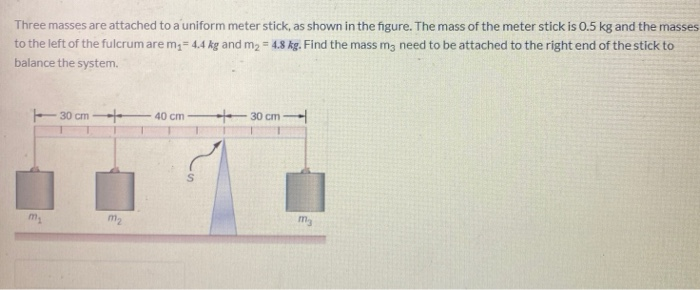Please help thank you! Three masses are attached to a uniform meter stick, as shown in the figure. The mass of the meter stick is 0.5 kg and the masses to the left of the fulcrum are m = 4.4 kg and m2 = 4.8 kg. Find the mass my need to be attached to the right end of the stick to balance the system - 30 cm 40 cm - 30 cm A wheel rotates without friction about a...

• ### A particle of mass 0.500 kg is attached to the 100-cm mark of a meter stick...

A particle of mass 0.500 kg is attached to the 100-cm mark of a meter stick of mass 0.200 kg. The meter stick rotates on a frictionless, horizontal table with an angular speed of 2.00 rad/s. (a) Calculate the angular momentum of the system when the stick is pivoted about an axis perpendicular to the table through the 50.0-cm mark. (b) Calculate the angular momentum of the system when the stick is pivoted about an axis perpendicular to the table...

• ### A uniform meter stick is supported on a knife edge at the 32.0 cm mark. When...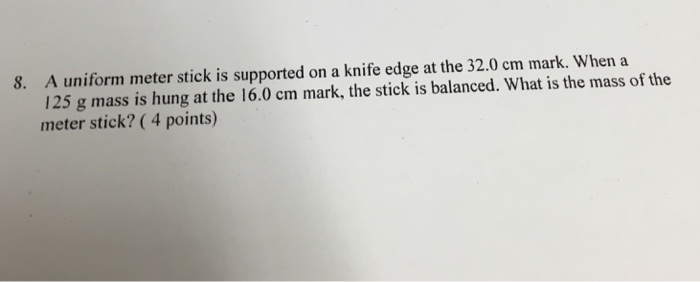A uniform meter stick is supported on a knife edge at the 32.0 cm mark. When a 125 g mass is hung at the 16.0 cm mark, the stick is balanced. What is the mass of the meter stick?

• ### A meter-stick is bolted to a wall. The bolt (pivot) is at the 5 cm mark....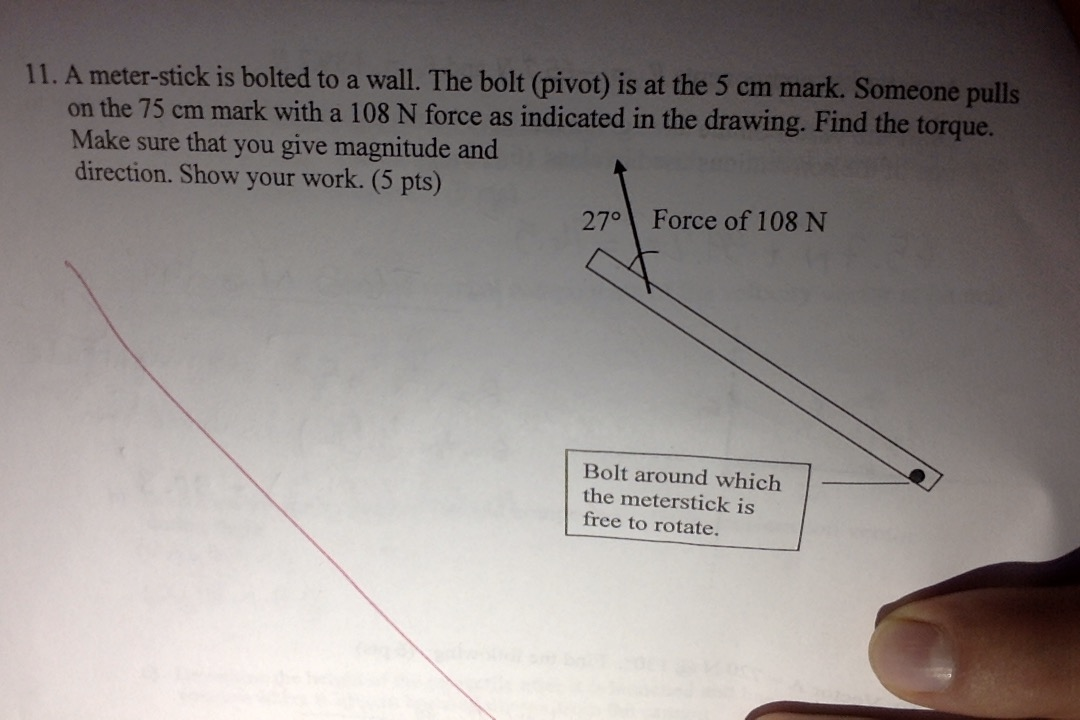A meter-stick is bolted to a wall. The bolt (pivot) is at the 5 cm mark. Someone pulls on the 75 cm mark with a 108 N force as indicated in the drawing. Find the torque . Give magnitude and direction. 11. A meter-stick is bolted to a wall. The bolt (pivot) is at the 5 cm mark. Someone pulls on the 75 cm mark with a 108 N force as indicated in the drawing. Find the torque. Make sure...

• ### A meter stick balances horizontally on a knife-edge at the 50.0 cm mark. With two 5.67...

A meter stick balances horizontally on a knife-edge at the 50.0 cm mark. With two 5.67 g coins stacked over the 36.0 cm mark, the stick is found to balance at the 42.1 cm mark. What is the mass of the meter stick?